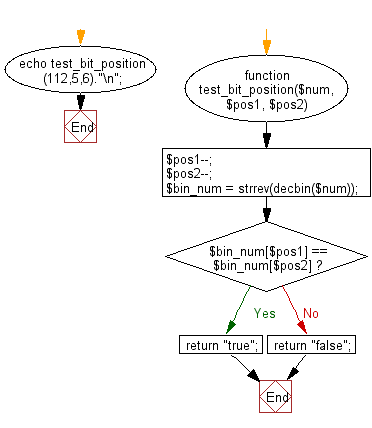﻿ PHP Exercise: Check if the bits of the two given positions of a number are same or not - w3resource# PHP Exercises: Check if the bits of the two given positions of a number are same or not

## PHP: Exercise-34 with Solution

Write a PHP program to check if the bits of the two given positions of a number are same or not.

112 - > 01110000
Test 2nd and 3rd position
Result: True
Test 4th and 5th position
Result: False

Sample Solution: -

PHP Code:

``````<?php
function test_bit_position(\$num, \$pos1, \$pos2) {
\$pos1--;
\$pos2--;
\$bin_num = strrev(decbin(\$num));
if (\$bin_num[\$pos1] == \$bin_num[\$pos2]) {
return "true";
} else {
return "false";
}
}
echo test_bit_position(112,5,6)."\n";
?>
```
```

Sample Output:

```true
```

Flowchart:PHP Code Editor:

Have another way to solve this solution? Contribute your code (and comments) through Disqus.

What is the difficulty level of this exercise?

﻿